# Jig:Saw’s puzzle solutions

## Puzzle 1

Later in the story, Zach and Milton go back to JIG:SAW and have to travel across the bridge again. When Zach reaches the discs of death, the population parameters of the red and blue discs are unchanged (Section 7.2.4), but he decides that the maximum speed he could tolerate is 45 rpm. Should he still choose the blue disc?

Looking back at Section 7.2.2, we can see that the population parameters for the red discs were m = 33, s = 8 and for the blue discs m = 45, s = 2. To find out the probability of the two disc colours spinning too fast for Zach to cope with, we first need to convert our score of 45 rpm to a z-score. For the red disc we’d get z = 1.5 and for the blue disc we’d get z = 0.

Red discs                                                Blue discs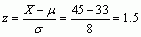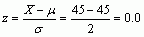We then need to look up the values of the corresponding zs in the table of the standard normal distribution. For the red disc, the corresponding z is positive (1.5), so we must read the value in the ‘smaller proportion’ column which is 0.06681. For the blue disc, the z score is also a positive value (0), so we must again read the value in the ‘smaller proportion’ column, which is 0.50000. So, there is a 6.68% chance that the red disc is one that spins at 45 rpm or faster, but a 50% chance for the blue disc. So, Zach should go for the red disc because the probability is smaller – it is less likely to be a disc that goes too fast for him to cope with.

## Puzzle 2

When Zach reaches the tunnels of death (Section 7.2.5) he decides that his previous estimate of being able to run through quicksand for 5 m was optimistic, so he reduces it to 4 m. The population parameters for the left and right tunnels are the same as before: which tunnel should he pick to maximize his chance of survival?

We need to calculate the probability that the length of each tunnel is 4 m or less, given the population from which it came. To do this we first need to convert the value of 4 m into a z-score for each tunnel. For the left tunnel we get z = −0.33 and for the right we get z = 0.

Left tunnel                                              Right tunnel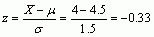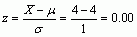We then need to look up the values of these corresponding zs in the table of the standard normal distribution. For the left tunnel, the corresponding z is negative (z = −0.33), so we must read the value in the ‘smaller proportion’ column, which is 0.37070. For the right tunnel the z score is positive (z = 0), so we must read the value in the ‘larger proportion’ column, which is o.50000. So, there is a 37.07% chance that the left tunnel is one that is 4 m long or less, and a 50% chance that the right tunnel is one that is 4m long or less. Therefore, Zach should choose the right tunnel.

## Puzzle 3

If the population parameters for the tunnels of death changed to be μ = 5 m, σ = 1.25 m for the left, and μ = 6 m, σ = 1.75 m for the right, and Zach still thought he could run through quicksand for 4 m, which tunnel should he choose?

As in puzzle 2, we need to calculate the probability that the length of each tunnel is 4 m or less, given the population from which it came. To do this we first need to convert the value of 4 m into a z-score for each tunnel. For the left tunnel we get z = −0.8 and for the right we get z = −1.14.

Left tunnel                                                Right tunnel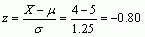Next we need to look up the values of these corresponding zs in the table of the standard normal distribution. For the left tunnel, because the corresponding z is negative (z = −0.8), we must read the value in the ‘smaller proportion’ column which is 0.21186. For the right tunnel, the z score is also negative (z = −1.14), so we must again read the value in the ‘smaller proportion’ column, which is 0.12714. So, there is a 21.19% chance that the left tunnel is one that is 4 m long or less, and a 12.71% chance that the right tunnel is one that is 4 m long or less. Therefore, Zach should choose the left tunnel this time.

## Puzzle 4

When Zach reaches the catapults of death (Section 7.2.6) the distance he needs to travel is still between 100 and 105 m, but the population parameters for the two catapults have changed. The left catapult comes from a population with μ = 101 m, σ = 5 m, and the right comes from population with μ = 105 m, σ = 3 m. Which catapult is most likely to propel him the safe distance of 100–105 m?

To find the probability that the left and right catapults will propel Zach safely between 100 and 105 m, we first need to convert the means and standard deviations of the two catapults into z-scores in the usual way.

Distance               Left catapult                               Right catapult

100°m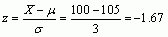105°mWe then need to look up the corresponding zs in the table of the standard normal distribution. For the left catapult, the smaller z is negative (z = −0.2), so we will note the value in the ‘smaller proportion’ column (see Section 7.2.4 and Zach’s Facts 7.3), which is o.42074. The larger z is positive (z = 0.8), so we will again note the value in the ‘smaller proportion’ column, which is 0.21186. The probability of the left catapult propelling Zach safely between 100 and 105 m is then 1 minus these two values, so we get 1 − 0.42074 − 0.21186 = 0.3674.

For the right catapult, the smaller z is positive (z = 0), so we will note the value in the ‘larger proportion’ column, which is 0.50000. The larger z is negative (z = −1.67), so we must note the value in the ‘smaller proportion’ column, which is 0.04746. The probability of the right catapult propelling Zach safely between 100 and 105 m is then 1 minus these two values, so we get 1 − 0.50000 − 0.04746 = 0.45254.

To sum up, there is a 36.74% chance that the left catapult will fire Zach between 100 and 105 meters and a 45.25% chance that the right catapult will fire Zach between 100 and 105 meters. Therefore, Zach should choose the right catapult.

## Puzzle 5

When Zach and Milton reach Deathscotch (Section 7.3), they see a different table of frequencies (Table 7.2 in the book, reproduced below). Should Zach still choose to hop onto a skull?

Table 7.2 (reproduced): A classification table of stones and outcomes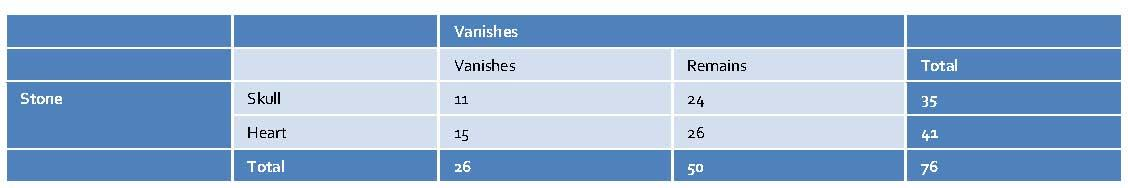To work out which stones are more likely to vanish, skulls or hearts, we need to use conditional probability. Conditional probability tells us the probability of an outcome, given that something else has happened. The ‘given that’ in the conditional probability statement restricts the probability space to a particular outcome. For example, if we want the probability of a stone vanishing given it’s a skull, we are restricting the probability space to skulls. In Table 7.2 we can see that there are 35 skulls, so that is our probability space, and of those 11 vanished, so the conditional probability is 11/35 (equation (1)). We can then calculate the probability of a stone vanishing, given it’s a heart, in the same way; in Table 7.2 we can see that there are 41 hearts, so that is our probability space, of which 15 vanished, so the conditional probability is 15/41 (equation (2)).(1)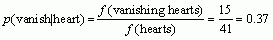(2)

So there is a probability of 0.31 that a skull will vanish and a probability of 0.37 that a heart will vanish.

We can reach the same conclusion by using probabilities rather than frequencies. The probability that a stone is a skull and vanishes is 11/76 because there are 76 stones in total and 11 of them are vanishing skulls, and the probability of a skull is 35/76 because there are 76 stones 35 of which are skulls. Both probabilities have 76 in the lower half of the fraction so the conditional probability reduces down to 11/35 = 0.31 or 31% (equation (3)). The probability that a stone is a heart and vanishes is 15/76 because there are 76 stones in total and 15 of them are vanishing hearts, and the probability of a heart is 41/76 because there are 76 stones 41 of which are hearts. Both probabilities have 76 in the lower half of the fraction so the conditional probability reduces down to 15/41 = 0.4 or 40% (equation (4)).(3)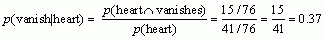(4)

A final way to calculate the conditional probability is using Bayes’ theorem: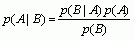For example, we could work out the conditional probability of a stone vanishing given it’s a skull,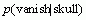, by knowing the probability of a skull:the probability of any stone vanishing: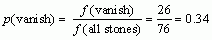and the reverse conditional probability, that is, the probability of a skull given that the stone vanished. There were 26 stones that vanished and 11 were skulls, so we get: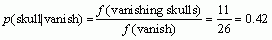Bayes’ theorem tells us that the conditional probability of the stone vanishing given you select a skull is: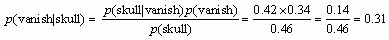So there is a 0.3 probability that a skull will vanish. We can work out the probability that a stone will vanish given it’s a heart in the same way: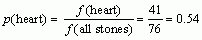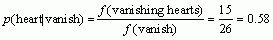Bayes’ theorem tells us that the conditional probability of the stone vanishing given you select a heart is: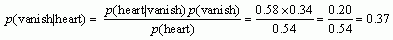Whichever of the three methods you use, there is a 0.37 probability that a heart will vanish and a 0.31 probability that a skull will vanish. Put another way, about 31% of skulls disappear and about 37% of hearts disappear, so Zach should still choose to jump onto a skull because hearts are still more likely to vanish than skulls.

## Puzzle 6

Using Table 6.1 (in the book), what is the probability that someone in the general population has a higher IQ than Zach? (Hint: use a value of IQ one more than Zach’s score.)

Zach’s IQ score was 125, so if we want to calculate the probability that someone in the general population has a score that is higher than this we can calculate the probability of someone having a score at least as big as 126 (one score higher than Zach’s). To do this we first need to convert 126 into a z-score using the population mean and standard deviation from Table 6.1, which were 100 and 15, respectively.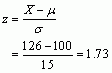To find the probability of obtaining a score at least as big as 126, we look up the value of the corresponding z, which is 1.73, in the table of the standard normal distribution. Because the z is positive, we need to read the value in the ‘smaller proportion’ column, which is 0.042, or 4.2%. Therefore, the probability of someone in the general population having an IQ score higher than Zach is 0.042.

## Puzzle 7

Using Table 6.1 (in the book), what is the probability that someone in the general population has as much creativity as Zach or more?

Zach’s creativity raw score was 30. To find the probability that someone in the general population has as much creativity as Zach or more, we need to look up the value of the corresponding z, which was 1.36 (see Table 6.1 in the book), in the table of the standard normal distribution. Because the z is positive we need to read the value in the ‘smaller proportion’ column, which is 0.087, or 8.7%. Therefore, the probability that someone in the general population has as much creativity as Zach or more is 0.087.

## Puzzle 8

Using Table 6.1 (in the book),, what is the probability that someone in the general population has the same emotional intelligence as Zach or lower?

Zach’s emotional intelligence raw score was 165. To find the probability that someone in the general population has the same amount of emotional intelligence as Zach or less, we need to look up the value of the corresponding z, which was 0.75 (see Table 6.1 in the book), in the table of the standard normal distribution. Because the z is positive we need to read the value in the ‘larger proportion’ column, which is 0.773 (or 77.3%). Therefore, the probability that someone in the general population has the same emotional intelligence as Zach or less is 0.773.

## Puzzle 9

Using Table 6.1 (in the book), what is the probability that a JIG:SAW code 1318 worker is at least as intelligent (IQ score) as Zach?

Zach’s IQ raw score was 125. To find the probability that a JIG:SAW code 1318 worker is at least as intelligent as Zach, we need to look up the value of Zach’s corresponding z (remember we’re looking at the z calculated using the code 1318 worker’s mean and standard deviation), which was –0.28 (see Table 6.1 in the book), in the table of the standard normal distribution. Because the z is negative we need to read the value in the ‘larger proportion’ column, which is 0.610, or 61%. Therefore, the probability that a JIG:SAW code 1318 worker is at least as intelligent as Zach is 0.61.

## Puzzle 10

Using Table 6.1 (in the book), what is the probability that a JIG:SAW technician is at least as intelligent (IQ score) as Zach?

Zach’s IQ raw score was 125. To find the probability that a JIG:SAW technician is at least as intelligent as Zach, we need to look up the value of Zach’s corresponding z, which was 0.5 (see Table 6.1 in the book; remember to look at the rows for JIG:SAW technicians), in the table of the standard normal distribution. Because the z is positive we need to read the value in the ‘smaller proportion’ column, which is 0.31, or 31%. Therefore, the probability that a JIG:SAW technician is at least as intelligent as Zach is 0.31.